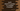# Swift program to check if a number is a multiple of another# Introduction :

We can check if a number is multiple of another number or not easily by division. If we divide the first number by the second number and if the remainder is 0, the second number is a multiple of the first number. But, swift also provides one method called isMultiple that we can use to find out if a number is multiple of another number or not. In this tutorial, we will learn how to use this method.

## Declaration of isMultiple :

The isMultiple method is declared as like below :

``func isMultiple(of other: Int) -> Bool``

As you can see here, it takes one argument other of type Int. It returns one boolean value defining if the caller integer is a multiple of others or not. true if yes and false otherwise.

Note that this method is available only for integer types. It is not available for any other types like Float or Double.

## Example :

Let’s try to understand isMultiple with an example :

``````import UIKit

print(4.isMultiple(of: 2))

print(44.isMultiple(of: 5))

print(12224.isMultiple(of: 20))``````

It will print :

``````true
false
false``````

## Find out the multiple of 5 below 100 using isMultiple :

We can also use isMultiple to find out the list of integers divisible by 5. You can use the same approach to find out integers divisible by any number x. Example program :

``````import UIKit

for n in 1...100{
if(n.isMultiple(of: 5)){
print(n)
}
}``````

It will give the below outputs :